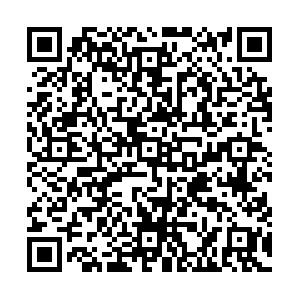# Phase structures of neutral dense quark matter and applicationto strange stars• In the contact interaction model, the quark propagator has only one solution, namely, the chiral symmetry breaking solution, at vanishing temperature and density in the case of physical quark mass. We generalize the condensate feedback onto the coupling strength from the 2 flavor case to the 2+1 flavor case, and find the Wigner solution appears in some regions, which enables us to tackle chiral phase transition as two-phase coexistences. At finite chemical potential, we analyze the chiral phase transition in the conditions of electric charge neutrality and $\beta$equilibrium. The four chemical potentials, $\mu_u$, $\mu_d$, $\mu_s$and $\mu_e$, are constrained by three conditions, so that one independent variable remains: we choose the average quark chemical potential as the free variable. All quark masses and number densities suffer discontinuities at the phase transition point. The strange quarks appear after the phase transition since the system needs more energy to produce a $d$-quark than an $s$-quark. Taking the EOS as an input, the TOV equations are solved numerically, and we show that the mass–radius relation is sensitive to the EOS. The maximum mass of strange quark stars is not susceptible to the parameter $\Lambda_q$we introduced.
••Get Citation
Shu-Sheng Xu. Phase structures of neutral dense quark matter and applicationto strange stars[J]. Chinese Physics C. doi: 10.1088/1674-1137/ac2f95
Shu-Sheng Xu. Phase structures of neutral dense quark matter and applicationto strange stars[J]. Chinese Physics C.Milestone
Article Metric

Article Views(17)
Cited by(0)
Policy on re-use
To reuse of Open Access content published by CPC, for content published under the terms of the Creative Commons Attribution 3.0 license (“CC CY”), the users don’t need to request permission to copy, distribute and display the final published version of the article and to create derivative works, subject to appropriate attribution.
###### 通讯作者: 陈斌, bchen63@163.com
• 1.

沈阳化工大学材料科学与工程学院 沈阳 110142

Title:
Email:

## Phase structures of neutral dense quark matter and applicationto strange stars

###### Corresponding author: Shu-Sheng Xu, xuss@njupt.edu.cn
• School of Science, Nanjing University of Posts and Telecommunications, Nanjing 210023, China

Abstract: In the contact interaction model, the quark propagator has only one solution, namely, the chiral symmetry breaking solution, at vanishing temperature and density in the case of physical quark mass. We generalize the condensate feedback onto the coupling strength from the 2 flavor case to the 2+1 flavor case, and find the Wigner solution appears in some regions, which enables us to tackle chiral phase transition as two-phase coexistences. At finite chemical potential, we analyze the chiral phase transition in the conditions of electric charge neutrality and $\beta$equilibrium. The four chemical potentials, $\mu_u$, $\mu_d$, $\mu_s$and $\mu_e$, are constrained by three conditions, so that one independent variable remains: we choose the average quark chemical potential as the free variable. All quark masses and number densities suffer discontinuities at the phase transition point. The strange quarks appear after the phase transition since the system needs more energy to produce a $d$-quark than an $s$-quark. Taking the EOS as an input, the TOV equations are solved numerically, and we show that the mass–radius relation is sensitive to the EOS. The maximum mass of strange quark stars is not susceptible to the parameter $\Lambda_q$we introduced.

### HTML关注分享DownLoad:  Full-Size Img  PowerPoint